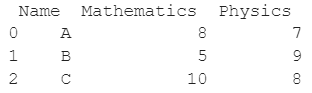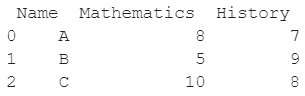# How to Rename Specific Column(s) In A Pandas DataFrame

A DataFrame is the primary data structure of the Pandas library in Python and is commonly used for storing and working with tabular data.

A common operation that could be performed on such data is to rename a column of an existing DataFrame in order to add more information to the data or to update already existing information.

To start working with Pandas, we first need to import pandas:

``import pandas as pd``

After importing, let us understand this operation with the help of an example.

Consider the following DataFrame containing 3 students with names A, B, and C and their corresponding marks (out of 10) for two subjects, Mathematics and Physics.Code snippet for generating the above DataFrame :

### Python 3 Code

``````import pandas as pd

# Dictionary for our data
data = {'Name' : ['A', 'B', 'C'], 'Mathematics' : [8, 5, 10], 'Physics' : [7, 9, 8]}

# DataFrame for the dictionary
df = pd.DataFrame(data)

# Printing the DataFrame
print(df)``````

Here, data is a dictionary that we created to initialize the DataFrame.

For this, we used the DataFrame() function of the Pandas library which takes the dictionary as an argument and returns the required DataFrame.

Now let us say that for some reason, we want to change the name of the column labeled as Physics to another subject, say History. The resulting DataFrame will look as follows :Let us look at different ways of performing this operation on a given DataFrame :

## Renaming Column By Using the DataFrame.rename() function

In this method, we shall use the DataFrame.rename() function to rename column names in an existing Pandas DataFrame.

The DataFrame.rename() function takes the first parameter as a python dictionary with the keys as original column names and corresponding values as new desired names, for example here the label of the third column has been changed from Physics to History.

The second parameter axis is set to 1 to specify that we need to work with the column axis. The third parameter inplace is set to True so that we do not need to reassign the DataFrame.

Let us look at the Python 3 code and corresponding output for this method:

``````import pandas as pd

# Dictionary for our data
data = {'Name' : ['A', 'B', 'C'], 'Mathematics' : [8, 5, 10], 'Physics' : [7, 9, 8]}

# DataFrame for the dictionary
df = pd.DataFrame(data)

# Setting the marks for Physics of the student named 'C' to 6
df.rename({'Physics':'History'}, axis = 1, inplace = True)

# Printing the resulting DataFrame
print(df)``````

Output :## Renaming Column by using the DataFrame.columns property

Here, we shall use the DataFrame.columns property to rename column names in an existing Pandas DataFrame.

The DataFrame.columns property returns a reference to a list containing the column labels of the DataFrame.

This list can be modified to contain the desired labels, for example here the label of the third column has been changed from Physics to History.

Let us look at the Python 3 code and corresponding output for this method:

``````import pandas as pd

# Dictionary for our data
data = {'Name' : ['A', 'B', 'C'], 'Mathematics' : [8, 5, 10], 'Physics' : [7, 9, 8]}

# DataFrame for the dictionary
df = pd.DataFrame(data)

# Setting the marks for Physics of the student named 'C' to 6
df.columns = ['Name', 'Mathematics', 'History']

# Printing the resulting DataFrame
print(df)``````

Output :However, if you want to change the column name by indexing the list returned by the DataFrame.columns property, it will throw an error.

Let us look at the code and produced an error for this alternate way as well:

``````import pandas as pd

# Dictionary for our data
data = {'Name' : ['A', 'B', 'C'], 'Mathematics' : [8, 5, 10], 'Physics' : [7, 9, 8]}

# DataFrame for the dictionary
df = pd.DataFrame(data)

# Setting the marks for Physics of the student named 'C' to 6
df.columns = 'History'

# Printing the resulting DataFrame
print(df)``````

Output :

TypeError: Index does not support mutable operations

## Renaming Column By using the DataFrame.set_axis() function

In this method, we shall use the DataFrame.set_axis() function to rename column names in an existing Pandas DataFrame.

The DataFrame.set_axis() function takes the first parameter as the list of new column labels of the DataFrame.

The second parameter axis is set to 1 to specify that we need to work with the column axis.

The third parameter inplace is set to True to specify that the updates need to be made in place so that there is no need for reassignment, for example here the label of the third column has been changed from Physics to History.

Let us look at the Python 3 code and corresponding output for this method:

``````import pandas as pd

# Dictionary for our data
data = {'Name' : ['A', 'B', 'C'], 'Mathematics' : [8, 5, 10], 'Physics' : [7, 9, 8]}

# DataFrame for the dictionary
df = pd.DataFrame(data)

# Setting the marks for Physics of the student named 'C' to 6
df.set_axis(['Name', 'Mathematics', 'History'], axis = 1, inplace = True)

# Printing the resulting DataFrame
print(df)``````

Output :## Conclusion

In this topic, we have learned to rename the column names of an existing Pandas DataFrame, following a running example of test scores of students in different subjects, thus giving us an intuition of how this concept could be applied in the real-world situations. Feel free to reach out to info.javaexercise@gmail.com in case of any suggestions.

Trending